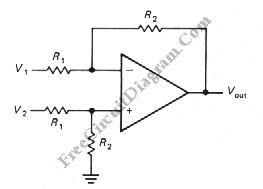## Complement Phase Inverter with Single Transistor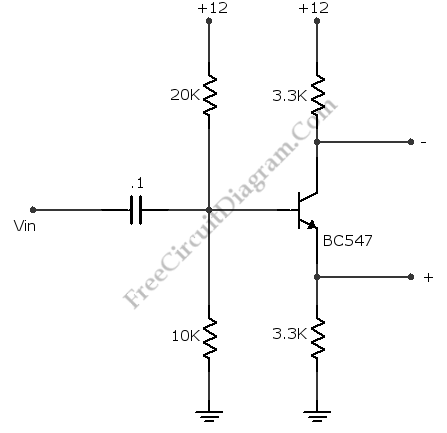This is a complement phase inverter circuit. Sometimes this circuit called a paraphase amplifier circuit. This circuit has two output signals that is have sufficient “headroom” because this circuit is biased. The transistor will be saturated when the collector decreases, the emitter voltage increases and set the limit. Here is the circuit: This circuit can produces a peak to peak […]

## 555 – 741 IC Voltage To Frequency Converter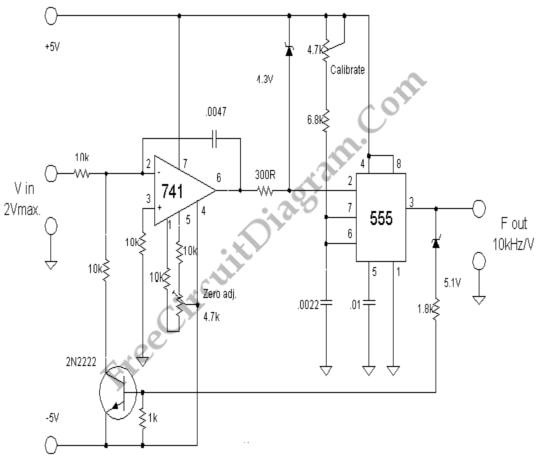This voltage to frequency converter (VFC) circuit uses 555 IC and 741 op-amp as the main components. Up to 20kHz oscillation can be produced by this circuit. Here is the schematic diagram: The zero adjustment is used to adjust the lowest frequency, short the input to ground and adjust this pot to obtain the lowest frequency limit. This circuit uses […]

## Low Distortion Adjustable Audio frequency Oscillator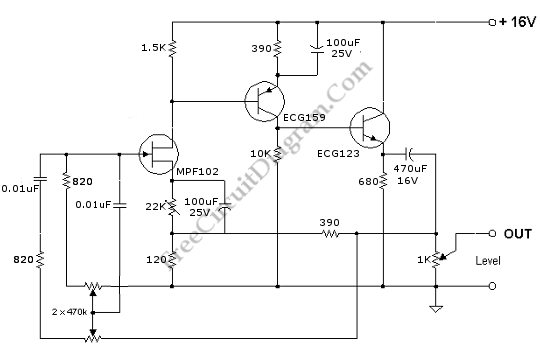Low distortion circuit is very important if we want to measure distortion level of an audio equipment, but this is just one example of low distortion oscillator circuit application. Here is the schematic diagram: This oscillator is designed for audio application, with the adjustable frequency range of 30Hz to 20kHz, set by 470k pot. This potentiometer should be of stereo […]

## Stable 60 Hz Frequency Signal Generator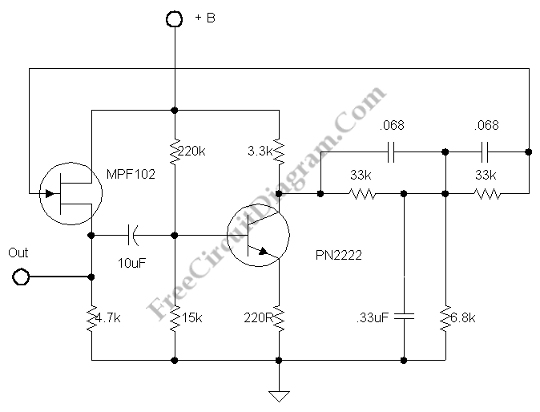A 60Hz signal generator can be useful in testing your circuits. You can test your notch filter, simulating hum noise, or you can use it as part of your DC-to-AC inverter design. Here is the schematic diagram of the circuit: The circuit consist of twin T network built around 33k resistors  and 0.068uF caps inside a feedback loop. You can […]

## Differentiator Operator with Op-Amp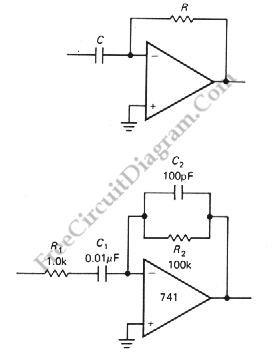Another application of OP-AMP is differentiator. Differentiator circuit produce an output if the input is changing. The output can positive or negative, depends on the change of the input: whether it’s decreasing or increasing.  This circuit is a bias stable. The current is produced by the rate of change of input voltage because the inverting input is grounded. Here is […]

## Summing Amplifier with Op-Amp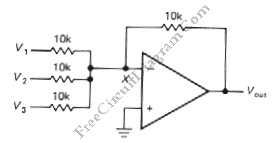One of inverting amplifier application is for summing several electric signals. The output of this circuit produce the sums of the input voltages.  We can use both positive or negative voltage for the inputs. The input resistors doesn’t have to be  same values. The input can be expanded to several digits. Since the point X is a virtual ground, the […]